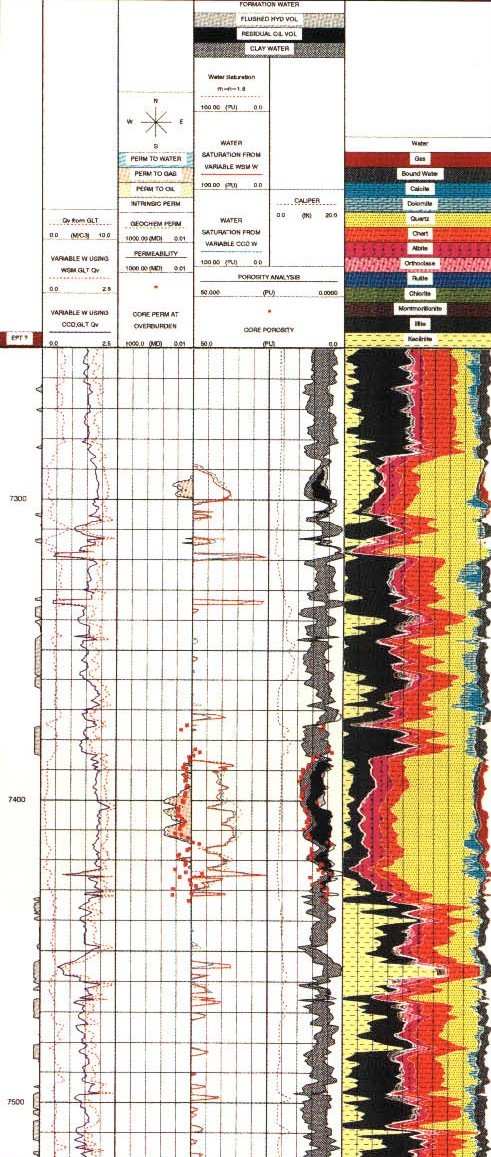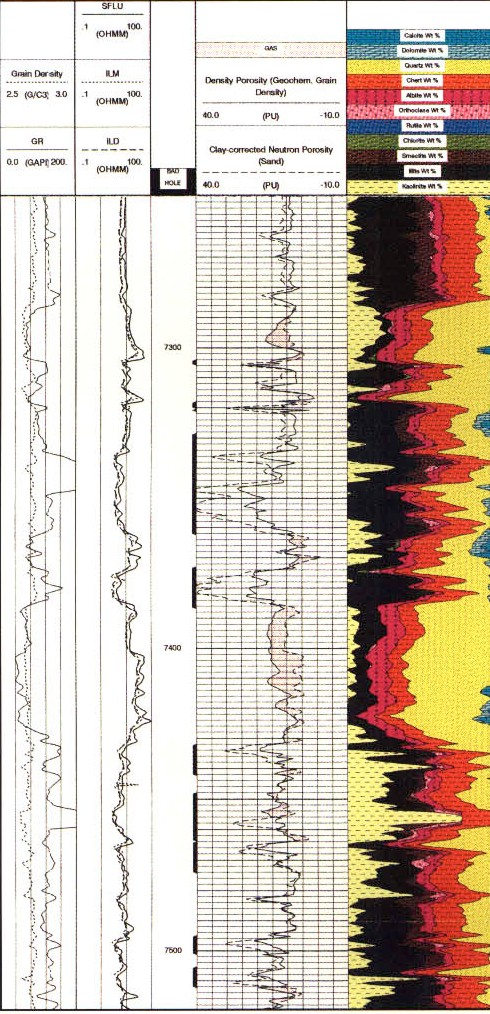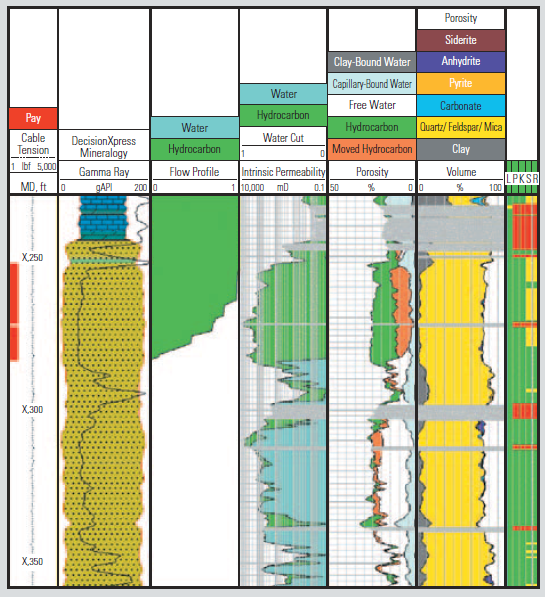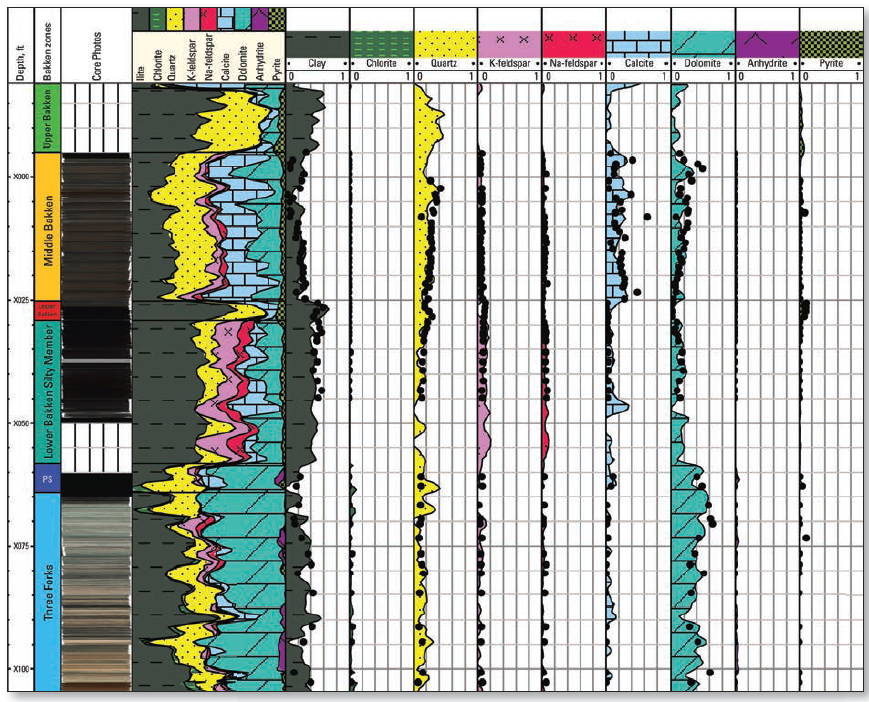CONVERTING ELEMENTS TO MINERALS
Induced gamma ray spectroscopy logs come in many flavours: geochemical log, elemental spectroscopy log, and many other descriptive trade names.

They are logs of elemental concentrations from which the mineral composition  of the formation may be derived.

The words "geochemical log" as used here should not be confused with the same term used to describe laboratory procedures to determine quite different chemical properties of  rocks.

Absolute mineral concentrations can be derived by using the oxide-closure model, which that defines what minerals are present. The first complete geochemical logs were run in the mid 1980s - the number of chemical elements that can be isolated has grown considerably since then.The oxide-closure model for converting relative elemental yields from a pulsed neutron spectroscopy log to absolute weight concentrations uses the assumption that the sum of all oxides in the rock matrix is 1.00. The model is based on the observation that, with few exceptions, sedimentary minerals are oxides, so that the sum of the dry weight percent of all oxides must be 100%. The weight percent of an oxide can be calculated from the dry weight percent of the cation by knowing the chemical formula.

Example of element yields from an induced GR spectroscopy log ==>

The absolute dry weight fraction, W, of element i is given by:
1:  Wi = F * Yi / Si

Where:
F = unknown normalization factor
Yi = measured spectral gamma ray yield
Si = tool sensitivity to that element, measured in the laboratory.

The dry weight fraction of the oxide is then:
2:Oi = F * Xi * Yi / Si

Where:
Oi = the oxide association factor, given by the chemical formula.

Since the sum of all Oi equals 1.00, it is possible to calculate F and determine each Wi .

The first step involves spectral deconvolution of the composite gamma ray energy spectrum by using a set of elemental standards to produce relative elemental yields. The relative yields are then converted to dry-weight elemental concentration logs for the elements.

For the ECS, the element list is  Si, Fe, Ca, S, Ti, and Gd using the oxides closure method. Matrix properties and quantitative dry-weight lithologies are then calculated from the dry-weight elemental fractions using  empirical relationships derived from an extensive core chemistry and mineralogy database.

The final display includes total clay, total carbonate,  anhydrite + gypsum, from, QFM (quartz + feldspar + mica),
pyrite, siderite, coal, and salt. Matrix properties, such as  matrix grain density, matrix thermal and epithermal neutron, and matrix sigma are also presented - helpful parameters for calculating porosity from other logs.

The LithoScamer uses inelastic and capture spectroscopy to detect 21 elemental yields including: Al, Ba, Br, C, Ca, Cl, Cu, Fe, Gd, H, K, Mg, Mn, Na, Ni, O, P, S, Si, Sr, Ti

These can be resolved into total organic carbon (TOC), anhydrite, clay, calcite, coal, dolomite, evaporite, pyrite, QFM, siderite. The metals copper, iron, magnesium, nickel, and aluminum can be identified in hard rock scenarios.

The Pulsar or advanced pulsed neutron log gives the results as shown above  and adds a new measurement, the fast neutron cross section (FNXS). This curve easily differentiates gas from oil and water.

This advanced tool is ideal for cased hole formation evaluation, especially in CO2, H2, and CH4 storage projects.EXAMPLE LOGSComputed lithology from oxide model, including porosity and hydrocarbon saturation from C/O ratio.Alternate analysis for lithology and chromostratigraphy.ECS Log showing volume track with mineral concentrations.LithoScanner mineralogy showing increased elemental precision:  carbonates separated into calcite and dolomite; chlorite from total clay.Pulsar Log:  simulated log response modeled in sand/shale sequence, comparing RHOB/TPHI with FNXS/TPHI overlays,

Page Views ---- Since 01 Jun 2022
Copyright 2023 by Accessible Petrophysics Ltd.
CPH Logo, "CPH", "CPH Gold Member", "CPH Platinum Member", "Crain's Rules", "Meta/Log", "Computer-Ready-Math", "Petro/Fusion Scripts" are Trademarks of the Author